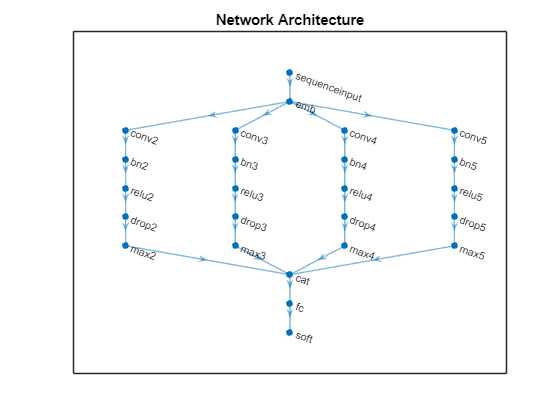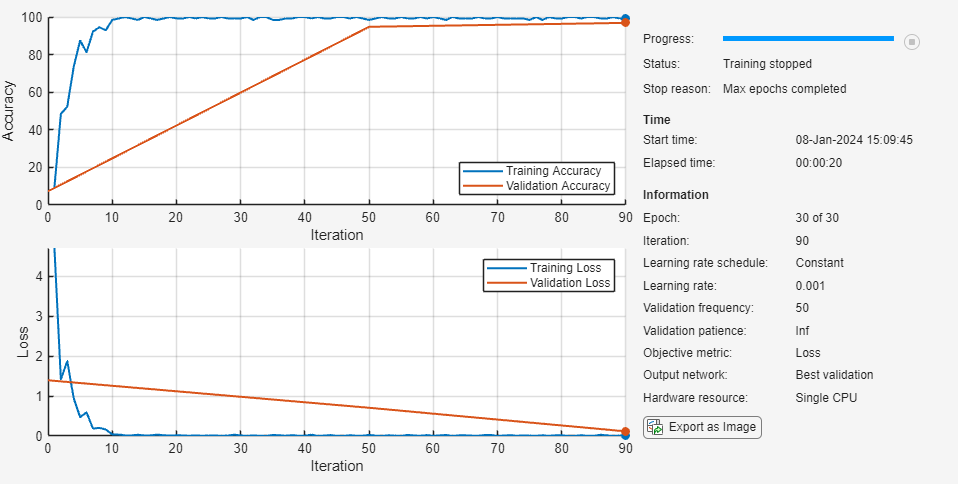Documentation

## Classify Text Data Using Convolutional Neural Network

This example shows how to classify text data using a convolutional neural network.

To classify text data using convolutions, you must convert the text data into images. To do this, pad or truncate the observations to have constant length S and convert the documents into sequences of word vectors of length C using a word embedding. You can then represent a document as a 1-by-S-by-C image (an image with height 1, width S, and C channels).

To convert text data from a CSV file to images, create a `tabularTextDatastore` object. The convert the data read from the `tabularTextDatastore` object to images for deep learning by calling `transform` with a custom transformation function. The `transformTextData` function, listed at the end of the example, takes data read from the datastore and a pretrained word embedding, and converts each observation to an array of word vectors.

This example trains a network with 1-D convolutional filters of varying widths. The width of each filter corresponds the number of words the filter can see (the n-gram length). The network has multiple branches of convolutional layers, so it can use different n-gram lengths.

### Load Pretrained Word Embedding

Load the pretrained fastText word embedding. This function requires the Text Analytics Toolbox™ Model for fastText English 16 Billion Token Word Embedding support package. If this support package is not installed, then the function provides a download link.

`emb = fastTextWordEmbedding;`

### Load Data

Create a tabular text datastore from the data in `weatherReportsTrain.csv`. Read the data from the `"event_narrative"` and `"event_type"` columns only.

```filenameTrain = "weatherReportsTrain.csv"; textName = "event_narrative"; labelName = "event_type"; ttdsTrain = tabularTextDatastore(filenameTrain,'SelectedVariableNames',[textName labelName]);```

Preview the datastore.

```ttdsTrain.ReadSize = 8; preview(ttdsTrain)```
```ans=8×2 table event_narrative event_type _________________________________________________________________________________________________________________________________________________________________________________________________ ___________________ 'Large tree down between Plantersville and Nettleton.' 'Thunderstorm Wind' 'One to two feet of deep standing water developed on a street on the Winthrop University campus after more than an inch of rain fell in less than an hour. One vehicle was stalled in the water.' 'Heavy Rain' 'NWS Columbia relayed a report of trees blown down along Tom Hall St.' 'Thunderstorm Wind' 'Media reported two trees blown down along I-40 in the Old Fort area.' 'Thunderstorm Wind' 'A few tree limbs greater than 6 inches down on HWY 18 in Roseland.' 'Thunderstorm Wind' 'Awning blown off a building on Lamar Avenue. Multiple trees down near the intersection of Winchester and Perkins.' 'Thunderstorm Wind' 'Tin roof ripped off house on Old Memphis Road near Billings Drive. Several large trees down in the area.' 'Thunderstorm Wind' 'Powerlines down at Walnut Grove and Cherry Lane roads.' 'Thunderstorm Wind' ```

Create a custom transform function that converts data read from the datastore to a table containing the predictors and the responses. The `transformTextData` function, listed at the end of the example, takes the data read from a `tabularTextDatastore` object and returns a table of predictors and responses. The predictors are 1-by-`sequenceLength`-by-C arrays of word vectors given by the word embedding `emb`, where C is the embedding dimension. The responses are categorical labels over the classes in `classNames`.

Read the labels from the training data using the `readLabels` function, listed at the end of the example, and find the unique class names.

```labels = readLabels(ttdsTrain,labelName); classNames = unique(labels); numObservations = numel(labels);```

Transform the datastore using `transformTextData` function and specify a sequence length of 100.

```sequenceLength = 100; tdsTrain = transform(ttdsTrain, @(data) transformTextData(data,sequenceLength,emb,classNames))```
```tdsTrain = TransformedDatastore with properties: UnderlyingDatastore: [1×1 matlab.io.datastore.TabularTextDatastore] Transforms: {@(data)transformTextData(data,sequenceLength,emb,classNames)} IncludeInfo: 0 ```

Preview the transformed datastore. The predictors are 1-by-S-by-C arrays, where S is the sequence length and C is the number of features (the embedding dimension). The responses are the categorical labels.

`preview(tdsTrain)`
```ans=8×2 table predictors responses __________________ _________________ [1×100×300 single] Thunderstorm Wind [1×100×300 single] Heavy Rain [1×100×300 single] Thunderstorm Wind [1×100×300 single] Thunderstorm Wind [1×100×300 single] Thunderstorm Wind [1×100×300 single] Thunderstorm Wind [1×100×300 single] Thunderstorm Wind [1×100×300 single] Thunderstorm Wind ```

Create a transformed datastore containing the validation data in `weatherReportsValidation.csv` using the same steps.

```filenameValidation = "weatherReportsValidation.csv"; ttdsValidation = tabularTextDatastore(filenameValidation,'SelectedVariableNames',[textName labelName]); tdsValidation = transform(ttdsValidation, @(data) transformTextData(data,sequenceLength,emb,classNames))```
```tdsValidation = TransformedDatastore with properties: UnderlyingDatastore: [1×1 matlab.io.datastore.TabularTextDatastore] Transforms: {@(data)transformTextData(data,sequenceLength,emb,classNames)} IncludeInfo: 0 ```

### Define Network Architecture

Define the network architecture for the classification task.

The following steps describe the network architecture.

• Specify an input size of 1-by-S-by-C, where S is the sequence length and C is the number of features (the embedding dimension).

• For the n-gram lengths 2, 3, 4, and 5, create blocks of layers containing a convolutional layer, a batch normalization layer, a ReLU layer, a dropout layer, and a max pooling layer.

• For each block, specify 200 convolutional filters of size 1-by-N and pooling regions of size 1-by-S, where N is the n-gram length.

• Connect the input layer to each block and concatenate the outputs of the blocks using a depth concatenation layer.

• To classify the outputs, include a fully connected layer with output size K, a softmax layer, and a classification layer, where K is the number of classes.

First, in a layer array, specify the input layer, the first block for unigrams, the depth concatenation layer, the fully connected layer, the softmax layer, and the classification layer.

```numFeatures = emb.Dimension; inputSize = [1 sequenceLength numFeatures]; numFilters = 200; ngramLengths = [2 3 4 5]; numBlocks = numel(ngramLengths); numClasses = numel(classNames);```

Create a layer graph containing the input layer. Set the normalization option to `'none'` and the layer name to `'input'`.

```layer = imageInputLayer(inputSize,'Normalization','none','Name','input'); lgraph = layerGraph(layer);```

For each of the n-gram lengths, create a block of convolution, batch normalization, ReLU, dropout, and max pooling layers. Connect each block to the input layer.

```for j = 1:numBlocks N = ngramLengths(j); block = [ convolution2dLayer([1 N],numFilters,'Name',"conv"+N,'Padding','same') batchNormalizationLayer('Name',"bn"+N) reluLayer('Name',"relu"+N) dropoutLayer(0.2,'Name',"drop"+N) maxPooling2dLayer([1 sequenceLength],'Name',"max"+N)]; lgraph = addLayers(lgraph,block); lgraph = connectLayers(lgraph,'input',"conv"+N); end```

View the network architecture in a plot.

```figure plot(lgraph) title("Network Architecture")```Add the depth concatenation layer, the fully connected layer, the softmax layer, and the classification layer.

```layers = [ depthConcatenationLayer(numBlocks,'Name','depth') fullyConnectedLayer(numClasses,'Name','fc') softmaxLayer('Name','soft') classificationLayer('Name','classification')]; lgraph = addLayers(lgraph,layers); figure plot(lgraph) title("Network Architecture")```Connect the max pooling layers to the depth concatenation layer and view the final network architecture in a plot.

```for j = 1:numBlocks N = ngramLengths(j); lgraph = connectLayers(lgraph,"max"+N,"depth/in"+j); end figure plot(lgraph) title("Network Architecture")```### Train Network

Specify the training options:

• Train for 10 epochs with a mini-batch size of 128.

• Validate the network at each epoch by setting the validation frequency to the number of iterations per epoch.

• Display the training progress plot and suppress the verbose output.

```miniBatchSize = 128; numIterationsPerEpoch = floor(numObservations/miniBatchSize); options = trainingOptions('adam', ... 'MaxEpochs',10, ... 'MiniBatchSize',miniBatchSize, ... 'ValidationData',tdsValidation, ... 'ValidationFrequency',numIterationsPerEpoch, ... 'Plots','training-progress', ... 'Verbose',false);```

Train the network using the `trainNetwork` function.

`net = trainNetwork(tdsTrain,lgraph,options);`### Test Network

Create a transformed datastore containing the held-out test data in `weatherReportsTest.csv`.

```filenameTest = "weatherReportsTest.csv"; ttdsTest = tabularTextDatastore(filenameTest,'SelectedVariableNames',[textName labelName]); tdsTest = transform(ttdsTest, @(data) transformTextData(data,sequenceLength,emb,classNames))```
```tdsTest = TransformedDatastore with properties: UnderlyingDatastore: [1×1 matlab.io.datastore.TabularTextDatastore] Transforms: {@(data)transformTextData(data,sequenceLength,emb,classNames)} IncludeInfo: 0 ```

Read the labels from the `tabularTextDatastore`.

```labelsTest = readLabels(ttdsTest,labelName); YTest = categorical(labelsTest,classNames);```

Make predictions on the test data using the trained network.

`YPred = classify(net,tdsTest);`

Calculate the classification accuracy on the test data.

`accuracy = mean(YPred == YTest)`
```accuracy = 0.8670 ```

### Functions

The `readLabels` function creates a copy of the `tabularTextDatastore` object `ttds` and reads the labels from the `labelName` column.

```function labels = readLabels(ttds,labelName) ttdsNew = copy(ttds); ttdsNew.SelectedVariableNames = labelName; tbl = readall(ttdsNew); labels = tbl.(labelName); end```

The `transformTextData` function takes the data read from a `tabularTextDatastore` object and returns a table of predictors and responses. The predictors are 1-by-`sequenceLength`-by-C arrays of word vectors given by the word embedding `emb`, where C is the embedding dimension. The responses are categorical labels over the classes in `classNames`.

```function dataTransformed = transformTextData(data,sequenceLength,emb,classNames) % Preprocess documents. textData = data{:,1}; textData = lower(textData); documents = tokenizedDocument(textData); % Convert documents to embeddingDimension-by-sequenceLength-by-1 images. predictors = doc2sequence(emb,documents,'Length',sequenceLength); % Reshape images to be of size 1-by-sequenceLength-embeddingDimension. predictors = cellfun(@(X) permute(X,[3 2 1]),predictors,'UniformOutput',false); % Read labels. labels = data{:,2}; responses = categorical(labels,classNames); % Convert data to table. dataTransformed = table(predictors,responses); end```